• 基于Java的GUI界面+SQL Server数据库的课程信息管理系统
• 成 C语言程序设计 绩 课程设计报告 专 业 班 级 姓 名 指导教师 二零一二年十二月 二十六日 目 录 一设计 目? 2 二设计 目的? 2 三设计内容 ? 2 3.1 需求分析 ?... 18 一设计题目课程信息管理系统 二设
• 课程信息管理系统 C语言版本 C语言课程设计 课程信息管理系统 C语言版本 C语言课程设计
• 课程信息管理系统 C语言程序 按老师要求，绝对正确完成
• 用vb简单编写的课程信息管理系统。基本功能已经实现。具体功能有待优化。2012.01.02
• 课程信息管理系统 课程信息包括：记录编号、课程编号、课程名、学时（讲课学时、实践学时）、学分、开课学期，考核方式（考试/报告） 功能要求： A、 从课程信息文件中读取已有的课程信息，并实现屏幕显示。 B、 能...
课程信息管理系统
课程信息包括：记录编号、课程编号、课程名、学时（讲课学时、实践学时）、学分、开课学期，考核方式（考试/报告）
功能要求：
A、	从课程信息文件中读取已有的课程信息，并实现屏幕显示。
B、	能通过课程编号对已有的课程信息进行修改，要求编号不能修改，其他信息内容都可改，修改的结果保存至课程信息文件。
C、	实现对课程信息的统计（分学期统计课程数量及开课总学分数）。
D、	实现对课程信息的查询显示（查询条件分为课程编号、课程学分、开课学期），对有多条记录的查询结果按照课程开课学期进行排序显示。
E、	新课程信息的添加并保存至课程信息文件。
F、	数据约束关系：学时=讲课学时+时间学时；学分=学时/16。
G、	课程信息的删除，根据课程编号，删除指定课程信息，并将结果写入文件。
本程序主要用到了类以及链表的知识。
后面代码中单链表及其功能的实现额解释在这个文章中
https://blog.csdn.net/xuese_luochen/article/details/104374323
头文件——Class.h
//头文件，用来定义类，并定义其成员函数
#ifndef CLASS_H_
#define CLASS_H_

#include <iostream>
#include <string>
#include <fstream>
using namespace std;

class Date						//类Date有数据成员，是课程的各种数据和一个类指针
{
public:
string name;
string exam_method;
int semester;
double time,number,credit;
Date *Classnext;
};

class Class						//类Class有成员函数——类指针（用来临时储存各个数据），和成员函数（用来实现程序的各种功能）
{
public:
Date *ClassHead;
~Class();							//析构函数
Class ();							//构造函数
void class_set ();					//输入课程信息
void show ();						//输出课程信息
void class_keep ();					//将课程信息写入文档
void class_insert(double N,string n,double t,int s,string e);		//将文档中的课程信息插入到链表中
void class_Read();					//从文档中读取课程信息
void class_delete();				//删除课程信息
void class_revise();				//修改课程信息
void class_sort();
void class_find();					//按照一定条件寻找课程
void class_renew();					//更新课程信息，并保持在文档中
void class_census();				//按学期统计课程数量和学分总数
};

Class::Class()              //构造函数
{
ClassHead=new Date;						//创建链表的链头
ClassHead->Classnext=NULL;
}

Class::~Class()             //析构函数
{
Date *p;
while (ClassHead)				//将链表清除
{
p=ClassHead;
ClassHead=ClassHead->Classnext;
delete p;
}
ClassHead=NULL;
}

void Class::class_set ()   //输入课程信息
{
class_sort();
Date *p;
Date *a;
int class_num;
a=ClassHead;
cout << "您要输入几个课程的信息" << endl;
cin >> class_num ;
cout << "请依次输入课程编号，课程名字，课程学时，课程开课学期，课程测试方式" << endl;
for(int k=0;k<class_num;k++)
{
p= new Date;
cin >> p->number >> p->name >> p->time >> p->semester >> p->exam_method ;
p->credit=p->time/16;					//下面三步实现将新的课程数据存放到链表中
p->Classnext=a->Classnext;
a->Classnext=p;
a=a->Classnext;
}
}

void Class::show ()                     //输出课程信息
{
Date *p;
cout << "课程编号" << '\t' << "课程名字" << '\t' << "课程学时" << '\t' << "课程学分" << '\t' << "课程学期" << '\t' << "课程考核方式" << endl;
for(p=ClassHead->Classnext;p!=NULL;p =p->Classnext)		//将链表各节点的数据全部输出
{
cout << p->number << '\t' << '\t' << p->name <<'\t' << p->time  <<'\t'  << '\t' << p->credit  <<'\t' << '\t' << p->semester << '\t' << '\t' << p->exam_method << endl;
}
class_sort();						//排序函数--方便程序排列
}

void Class::class_find()				//查找课程
{
Date *p;
p=ClassHead;
int m,k,a=1;			//a的作用是①如能查到则只输出一次提示②如不能查到则输出提示语
cout << "通过课程序号进行查询请按1，通过课程学期请按2，通过学分查询请按3,退出请按0" << endl;
cin >> m;
if(m==1)													//按课程编号查询
{
cout << "请输入您要查询课程的编号" << endl;
cin >> k;
while (p->Classnext && p->number!=k)
{
p=p->Classnext;
}
if(p->number==k)
{
if(a==1)   cout << "课程编号" << '\t' << "课程名字" << '\t' << "课程学时" << '\t' << "课程学分" << '\t' << "课程学期" << '\t' << "课程考核方式" << endl;
cout << p->number << '\t' << '\t' << p->name <<'\t' << p->time  <<'\t'  << '\t' << p->credit  <<'\t' << '\t' << p->semester << '\t' << '\t' << p->exam_method << endl;
a++;
}
else
{
cout << "未查询到课程" << endl;
}
}
else if(m==2)												//按学期查询
{
cout << "请输入您要查询第几学期的课程" << endl;
cin >> k;
while(p!=NULL)
{
if(p->semester==k)
{
if(a==1) cout << "课程编号" << '\t' << "课程名字" << '\t' << "课程学时" << '\t' << "课程学分" << '\t' << "课程学期" << '\t' << "课程考核方式" << endl;
cout << p->number << '\t' << '\t' << p->name <<'\t' << p->time  <<'\t'  << '\t' << p->credit  <<'\t' << '\t' << p->semester << '\t' << '\t' << p->exam_method << endl;
a++;
}
p=p->Classnext;
}
if(a==1)  cout << "未查询到课程" << endl;
}
else if(m==3)											//按学分查询
{
cout << "请输入您要查询学分为几的课程" << endl;
cin >> k;
while(p!=NULL)
{
if(p->credit==k)
{
if(a==1) cout << "课程编号" << '\t' << "课程名字" << '\t' << "课程学时" << '\t' << "课程学分" << '\t' << "课程学期" << '\t' << "课程考核方式" << endl;
cout << p->number << '\t' << '\t' << p->name <<'\t' << p->time  <<'\t'  << '\t' << p->credit  <<'\t' << '\t' << p->semester << '\t' << '\t' << p->exam_method << endl;
a++;
}
p=p->Classnext;
}
if(a==1)  cout << "未查询到课程" << endl;;
}
else cout << "请输入正确的指令" << endl;
}

void Class::class_delete()					//删除某个课程
{
int k;
Date *p,*a;
p=ClassHead;
a=p;
cout << "请输入您要删除课程的序号" << endl;
cin >> k;
while (p->Classnext && p->number!=k)
{
a=p;
p=p->Classnext;
}
if(p->number==k)
{
a->Classnext=p->Classnext;
delete p;
}
else
{
cout << "未查询到课程" << endl;
}
}

void Class::class_sort()					//对课程进行排序（按编号大小）
{
string n;
string e;
int s,m=0,j,i;
double t,N,c;
Date *p1,*p2;
p1=ClassHead;
while(p1->Classnext!=NULL)
{
p1=p1->Classnext;
m++;
}
for(i=0;i<m-i;i++)
{
p2=ClassHead->Classnext;
p1=p2->Classnext;
for(j=0;j< m-i-1;j++)
{
if(p1->number >p2->number)
{
N=p1->number;
n=p1->name;
t=p1->time;
c=p1->credit;
s=p1->semester;
e=p1->exam_method;
p1->number = p2->number;
p1->name = p2->name;
p1->time = p2->time;
p1->credit = p2->credit;
p1->semester = p2->semester;
p1->exam_method = p2->exam_method;
p2->number=N;
p2->name=n;
p2->time=t;
p2->credit=c;
p2->semester=s;
p2->exam_method=e;
}
p1=p1->Classnext;
p2=p2->Classnext;
}
}
}

void Class::class_revise()						//修改课程数据
{
int k;
cout << "请输入您要修改课程的编号" << endl;
cin >> k;
Date *p;
p=ClassHead;
while(p->Classnext && p->number!=k)
{
p=p->Classnext;
}
if(p->number==k)
{
cout << "请重新输入该课程信息（分别为课程名字，课程学时，课程学期，课程考核方式）" << endl;
cin  >> p->name >> p->time >> p->semester >> p->exam_method ;
p->credit=p->time/16;
cout << "修改完成" << endl;
}
else cout<< "对不起，没有查询到课程，请输入已经存在的课程编号" <<endl;
}

void Class::class_insert(double N,string n,double t,int s,string e)			//将文件中的数课程据插入到链表中
{
Date *a;
Date *p;
a=ClassHead;
p=new Date;
p->number=N;
p->name=n;
p->time=t;
p->credit=t/16;
p->semester=s;
p->exam_method=e;
p->Classnext=a->Classnext;
a->Classnext=p;
}

void Class::class_census()							//按学期来统计课程数目及学分总数
{
double a=0,b=0,k;
Date *p;
p=ClassHead;
cout << "您要查看那个学期的课程统计？" << endl;
cin >> k ;
while(p!=NULL)
{
if(p->semester==k)
{
a++;				//课程数目
b=b+p->credit;
}
p=p->Classnext;			//课程总学分
}
cout << "第" << k << "学期共有" << a << "门课程，总学分为" << b << endl;
}

void Class::class_keep ()    //保存到文件里面
{
class_sort();
Date *p;
p=ClassHead->Classnext;
ofstream out("class.txt",ios::app);					//打开文件，在文件class.txt的结尾处进行添加数据
if (!out) { cout << "不能打开文件！" << endl; return; }
while (p != NULL)
{
out << p->number << '\t' << p->name << '\t' << p->time  << '\t' << p->semester <<  '\t' << p->exam_method << '\t' << endl;
p = p->Classnext;
}
out.close();										//关闭文件
}

void Class::class_renew ()    //将文件覆盖（更新）
{
Date *p;
p=ClassHead->Classnext;
ofstream out("class.txt");							//打开文件，直接覆盖原有数据
if (!out) { cout << "不能打开文件！" << endl; return; }
while (p != NULL)
{
out << p->number << '\t' << p->name << '\t' << p->time  << '\t' << p->semester <<  '\t' << p->exam_method << '\t' << endl;
p = p->Classnext;
}
out.close();										//关闭文件
}

void Class::class_Read()    //从文件读入数据
{
int a=0;
Date *p;
p = ClassHead;
ifstream in;
in.open("class.txt");								//打开文件
if (!in) { cout << "没有课程信息，请先录入课程信息!" << endl; return; }
string n,e;
int s;
double N,t;
while(!in.eof())
{
in >> N >> n >> t >> s >> e;
if(in.fail()) break;
class_insert(N,n,t,s,e);
}
in.close();											//关闭文件
}

int door()
{
string username = "123", password = "123";
string name, temp;
int number = 3;
while (1)
{
cout << "         欢迎登陆家里蹲抗大大学教务系统  "<< endl;
cout << endl;
cout << "                用 户 名：";
cin >> name;
cout << "                密    码：";
cin >> temp;
if (name != username || temp != password)
{
number--;
if (number >0)
{
cout << "          用户名/密码错误!你还有" << number << "次机会" << endl;
}
else
cout << "用户名/密码错误!" << endl, exit(0);

}
else
{
cout << "********************密码正确********************" << endl<<endl;
return 1;
}
}

}

void menu ()
{
cout << "                    ----------------------------------------" <<endl;
cout << "                    |                                     | " <<endl;
cout << "                    |     欢迎使用本课程信息管理系统      | " <<endl;
cout << "                    |     如需查看已有课程请按  1         | " <<endl;
cout << "                    |     如需查找课程请按      2         | " <<endl;
cout << "                    |     如需添加新课程请按    3         | " <<endl;
cout << "                    |     如需修改课程信息请按  4         | " <<endl;
cout << "                    |     如需删除课程请按      5         | " <<endl;
cout << "                    |     如需导入课程请按      6		   | " <<endl;
cout << "                    |     如需统计学期课程请按  7         | " <<endl;
cout << "                    |     如需退出系统请按      0         | " <<endl;
cout << "                    |                                     | " <<endl;
cout << "                    ----------------------------------------" <<endl;
}

#endif;

头文件有个小问题：在输入课程信息时课程名字只有在输入四个字时才会对齐，否则会偏,如果哪位大佬会就顺便教一下吧（手动滑稽）。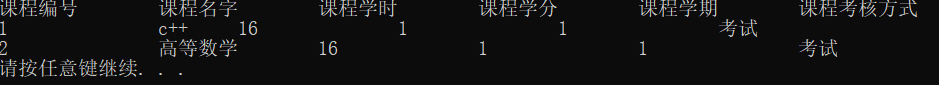主文件
//本程序为课程信息管理系统
#include <iostream>
#include "Class.h"
#include<cstdlib>
#include <String>
using namespace std;

int main()
{
door();
int k;
string m;
Class c;
while(1)
{
system("cls");
menu();
cout << "请问您要执行哪一个功能呢，请输入相应数字" << endl;
cin >> k;
switch(k)
{
case 1:
c.class_sort();
c.show();
system("pause");
break;
case 2:
c.class_find();
system("pause");
break;
case 3:
c.class_set();
c.class_keep();
cout << "课程输出完成，现有课程如下" << endl;
c.show();
system("pause");
break;
case 4:
cout <<  "现有课程如下:" << endl;
c.show();
c.class_revise();
cout << "更新成功，现有课程如下:" << endl;
c.show();
c.class_renew();
system("pause");
break;
case 5:
cout <<  "现有课程如下:" << endl;
c.show();
c.class_delete();
cout << "更新成功，现有课程如下:" << endl;
c.show();
c.class_renew();
system("pause");
break;
case 6:
c.class_Read();
c.show();
system("pause");
break;
case 7:
c.class_census();
system("pause");
break;
case 0:
cout << "感谢您的使用" << endl;
exit(0);
}
}
return 0;
}




展开全文c++ 单链表
• 1748741328，基于jsp+servlet的课程信息管理系统，登录界面，如下所示： 2、基于jsp+servlet的课程信息管理系统，注册界面，如下所示： 3、基于jsp+servlet的课程信息管理系统，系统主界面，如下所示： 4、...
运行环境，jdk1.8或者jdk1.7、tomcat8或者tomcat8.5、mysql5.7、eclipse、myeclipse开发环境。

1、🐧1748741328，基于jsp+servlet的课程信息管理系统，登录界面，如下所示：2、基于jsp+servlet的课程信息管理系统，注册界面，如下所示：3、基于jsp+servlet的课程信息管理系统，系统主界面，如下所示：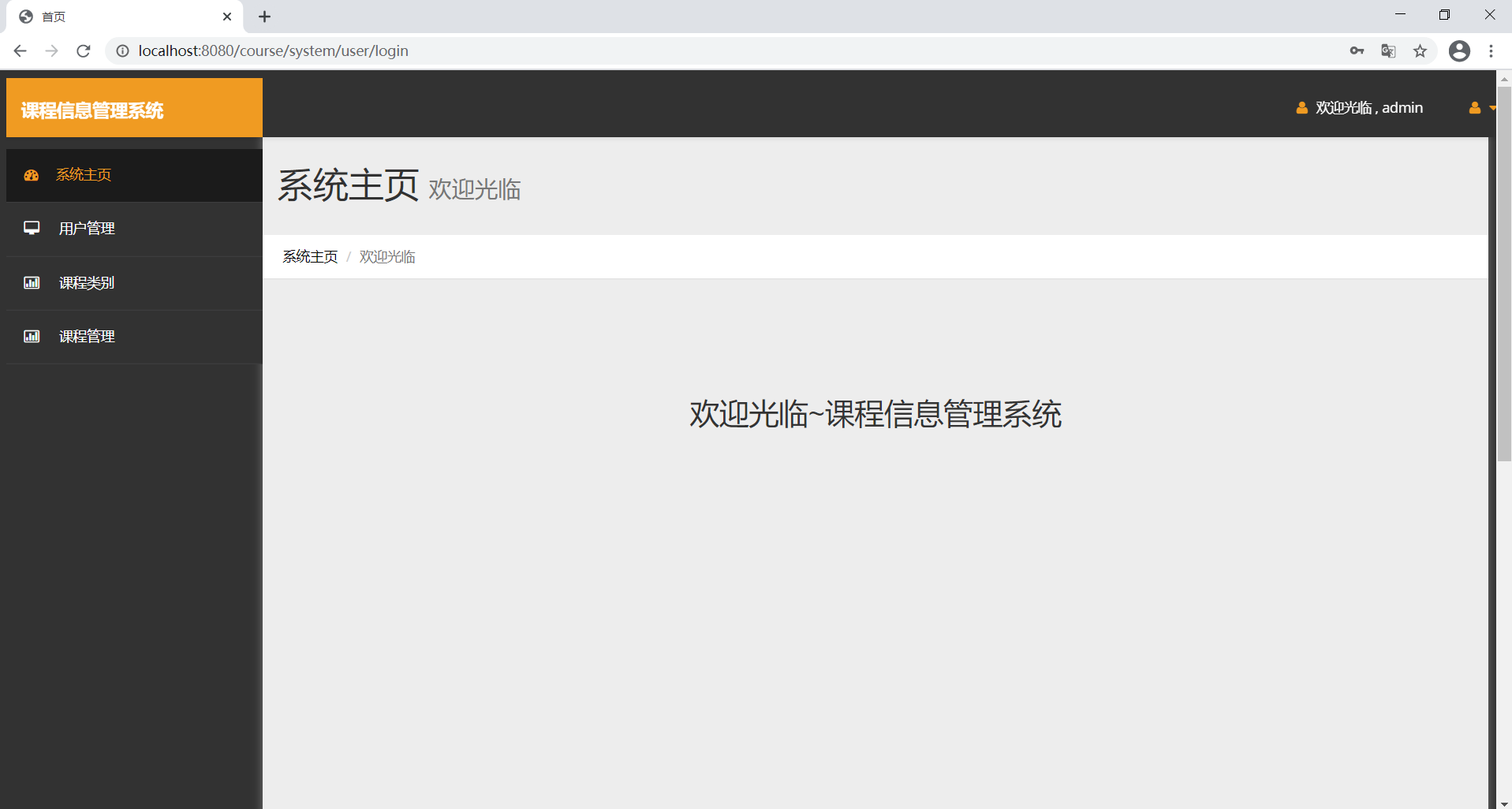4、基于jsp+servlet的课程信息管理系统，用户管理，如下所示：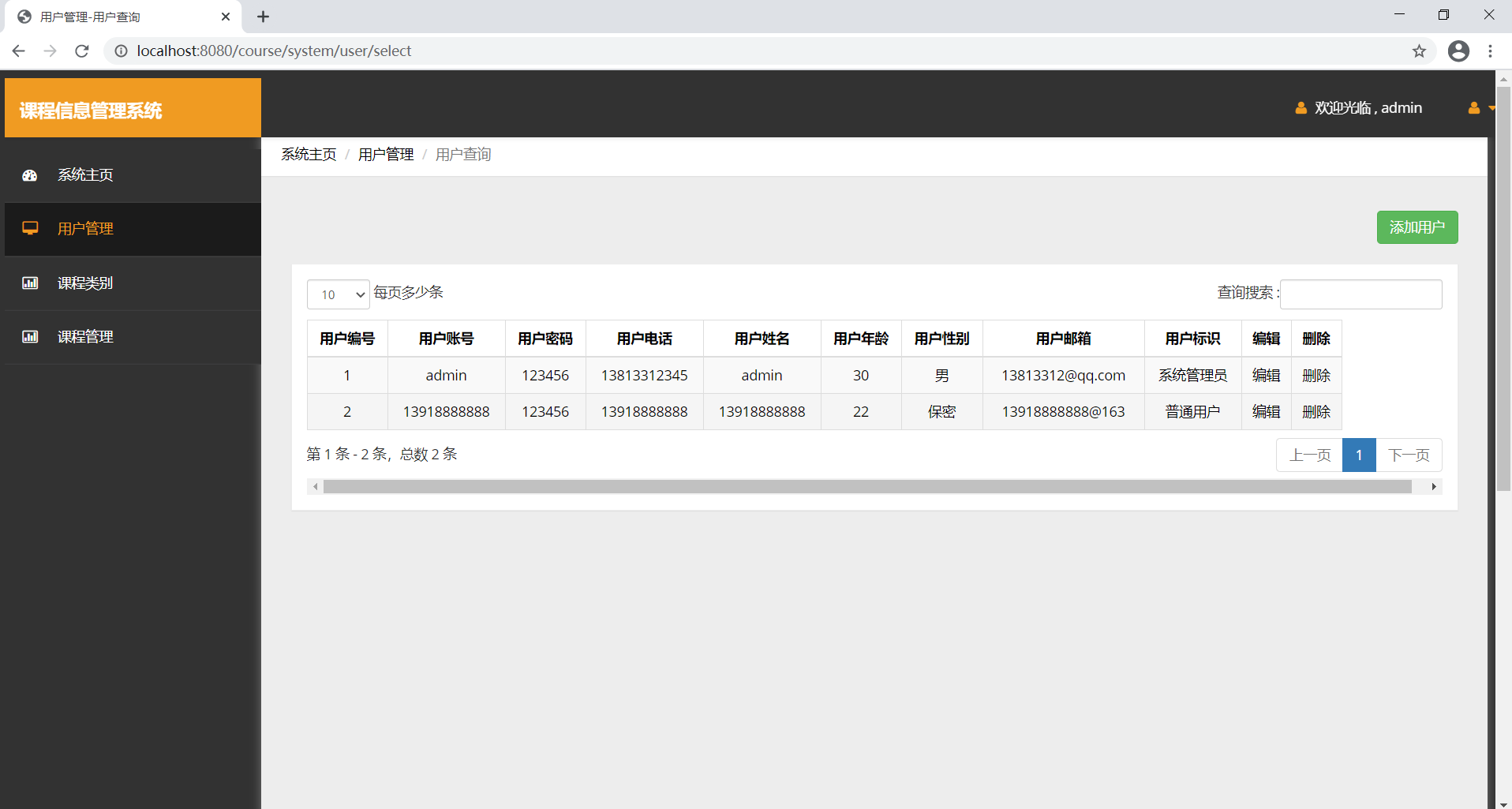5、基于jsp+servlet的课程信息管理系统，课程类别管理，如下所示：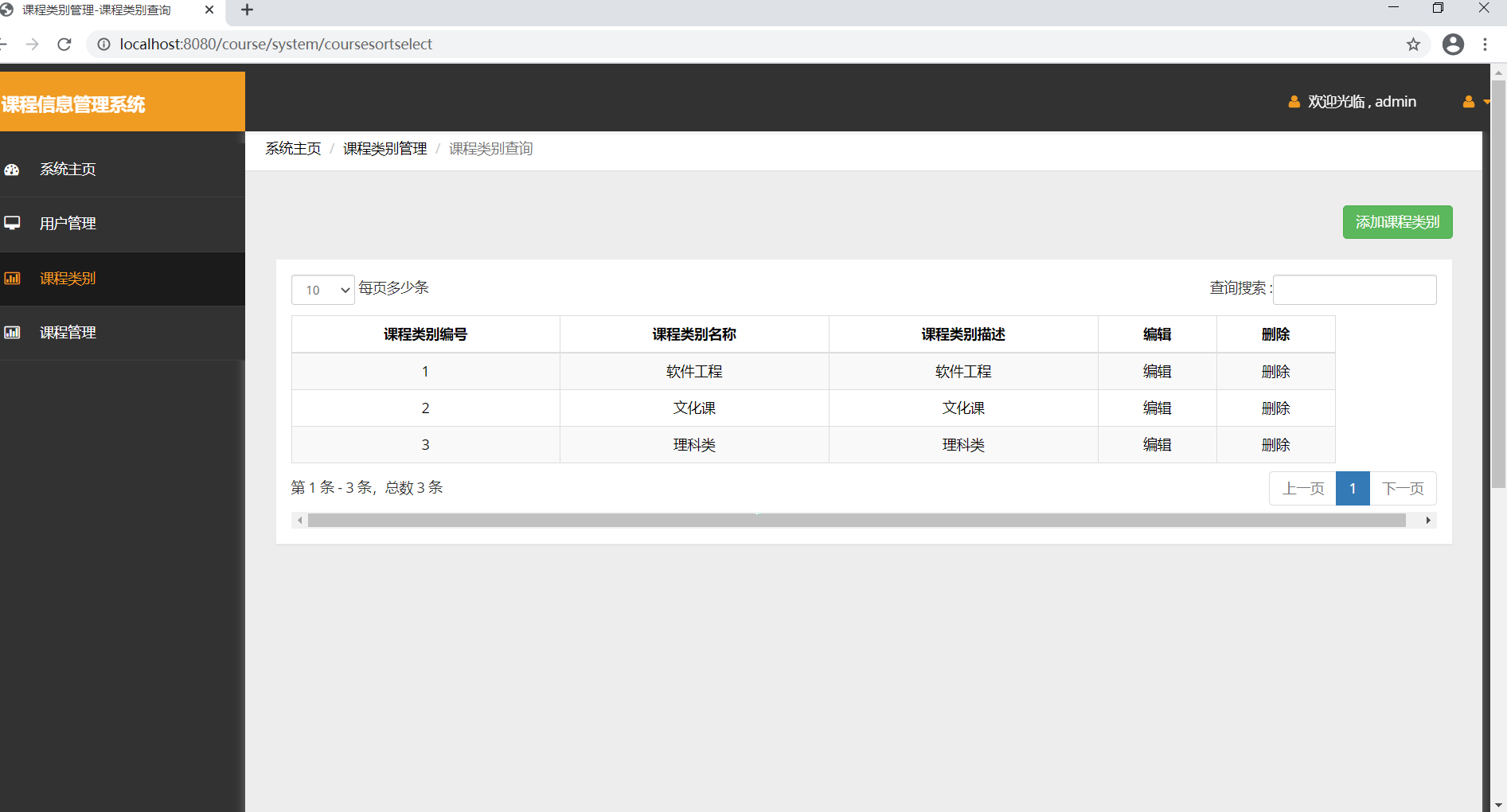6、基于jsp+servlet的课程信息管理系统，课程管理，如下所示：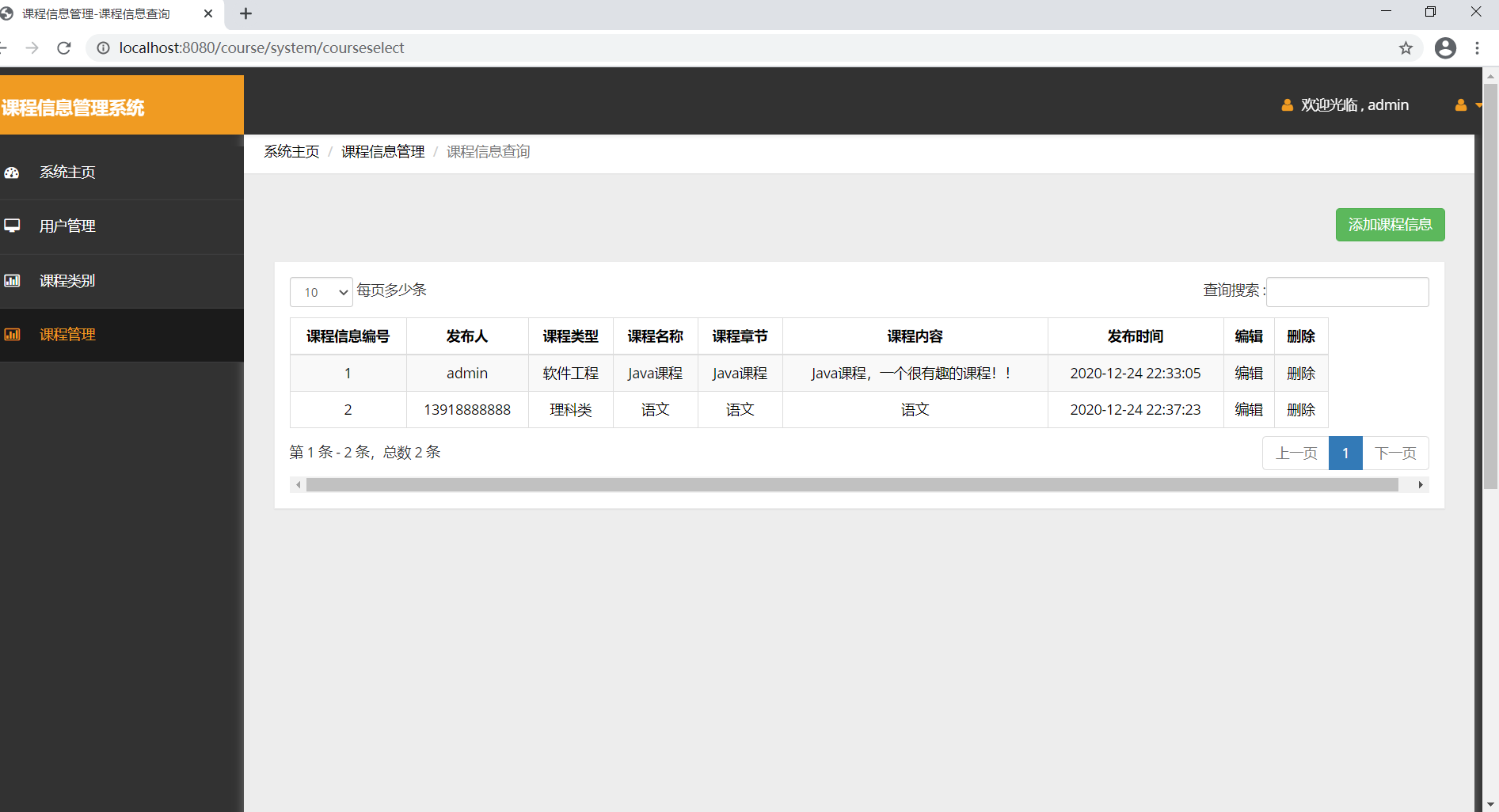展开全文jsp servlet
• 课程信息管理系统包括对课程信息的添加，修改，删除，打印，排序等功能。在登录管理员系统时的会询问密码，密码为ajaxhe。
• 本实例介绍如何构造一个ODBC数据库以及通过MFC如何使用ODBC数据库，并且通过MFC来实现一个课程信息管理系统。用vc6.0编写
• 自己有VC++MFC做的一个简单的课程信息管理系统 基本功能都具备 还有右键关联 但删除有点问题
• Web开发之MVC模式实现学生课程信息管理系统 什么是mvc模式？ MVC是一个架构，或者说是一个设计模式，它就是强制性使应用程序的输入，处理和输出分开。将一个应用程序分为三个部分：Model，View，Controller。 ...
Web开发之MVC模式实现学生课程信息管理系统
什么是mvc模式？
MVC是一个架构，或者说是一个设计模式，它就是强制性使应用程序的输入，处理和输出分开。将一个应用程序分为三个部分：Model，View，Controller。
Model模型（完成业务逻辑：由javaBean构成，在MVC的三个部件中，模型拥有最多的处理任务。例如它可能用象EJBs和javabean这样的构件对象来处理数据库。由于应用于模型的代码只需写一次就可以被多个视图重用，所以减少了代码的重复性。）
View 视图（就是负责跟用户交互的界面。一般就是由HTML，css元素组成的界面，当然现在还有一些像js，ajax，flex一些也都属于视图层。 在视图层里没有真正的处理发生，之负责数据输出，并允许用户操纵的方式。MVC能为应用程序处理很多不同的视图。 ）
Controller 控制器（接收请求—>调用模型—>根据结果派发页面并经过模型处理返回相应数据 ）
Mvc模式的优缺点：
优点：
1、分工明确（开发人员可以只关注整个结构中的其中某一层）：使用MVC可以把数据库开发，程序业务逻辑开发，页面开发分开，每一层都具有相同的特征，方便以后的代码维护。
它使程序员（Java开发人员）集中精力于业务逻辑，界面程序员（HTML和JSP开发人员）集中精力于表现形式上。
2、松耦合（可以降低层与层之间的依赖）：视图层和业务层分离，这样就允许更改视图层代码而不用重新编译模型和控制器代码，同样，一个应用的业务流程或者业务规则的改变只需要改动MVC的模型层即可。因为模型与控制器和视图相分离，所以很容易改变应用程序的数据层和业务规则。
3、复用性高（利于各层逻辑的复用）：像多个视图能够共享一个模型，不论你视图层是用flash界面或是wap界面，用一个模型就能处理他们。将数据和业务规则从表示层分开，就可以最大化从用代码。、
3、有利于标准化（有利于通过工程化、工具化产生管理程序代码）；
用更通俗易懂的话来说就是：分层式设计可以达到：分散关注、松散耦合、逻辑复用、标准定义的目的。
缺点：
1、有时会导致级联的修改。这种修改尤其体现在自上而下的方向。如果在表示层中需要增加一个功能，为保证其设计符合分层式结构，可能需要在相应的业务逻辑层和数据访问层中都增加相应的代码。
2、降低了系统的性能。这是不言而喻的。如果不采用分层式结构，很多业务可以直接造访数据库，以此获取相应的数据，如今却必须通过中间层来完成。
3、由于它没有明确的定义，所以完全理解MVC并不是很容易。使用MVC需要精心的计划，由于它的内部原理比较复杂，所以需要花费一些时间去思考。
4、MVC并不适合小型甚至中等规模的应用程序，花费大量时间将MVC应用到规模并不是很大的应用程序通常会得不偿失。
下面是我用javabean+jsp+servlet来实现一个实现一个简单的web项目—一个简易的学生课程信息的管理系统。
其中：
JavaBean对应于Model层
Jsp对应于View层
Servlet对应于Controller层
这三者之间的调用关系如下：
学生课程管理系统能够实现学生课程信息的增删改查功能：
系统功能的具体实现：
For example：
查找学生课程信息功能的实现：
写一个jsp页面，包含一个标签，点击链接到courseFindservlet。courseFindserrvlet用于接受请求， 然后调用JavaBean，由JavaBean来与数据库进行数据的交互，完成数据交互后，然后courseFindservlet将数据转发给jsp页面，jsp页面最后响应浏览器的请求，将学生课程的信息展示在浏览器上，查询学生课程相关信息的功能就实现了。
增加学生课程信息功能的实现：
写一个jsp页面，然后设置一个添加按钮，点击跳转到添加课程信息的一个界面，然后写一个添加信息的界面，包含一个标签，点击链接到增加课程信息的courseAddservlet。这个servlet用于接受请求，然后调用JavaBean，由JavaBean来与数据库进行数据的交互，将数据存储到后台的数据库中，然后将数据转发给jsp页面，点击提交按钮后跳转到学生课程信息的jsp页面，jsp页面最后响应浏览器的请求，显示学生课程信息，发现添加成功，添加学生课程相关信息的功能就实现了。
其他功能的实现大体一致，就不一一展示了。
项目的目录如下：
Jsp文件如下：
项目部分重要代码如下：
课程实体类代码：
public class Course1 {
private String cid;
public String cxf;
public String cmax;
public String cgrade;
public String ctea;
public String cname;
public String getCid() {
return cid;
}

public void setCid(String cid) {
this.cid = cid;
}
public String getCxf() {
return cxf;
}

public void setCxf(String cxf) {
this.cxf = cxf;
}
public String getCmax() {
return cmax;
}

public void setCmax(String cmax) {
this.cmax= cmax;
}
public String getCgrade() {
return cgrade;
}

public void setCgrade(String cgrade) {
this.cgrade = cgrade;
}
public String getCtea() {
return ctea;
}

public void setCtea(String ctea) {
this.ctea = ctea;
}
public String getCname() {
return cname;
}

public void setCname(String cname) {
this.cname = cname;
}

添加课程的具体函数如下：
添加功能实现的servlet的主要代码：
protected void doPost(HttpServletRequest req, HttpServletResponse resp) throws ServletException, IOException {
String couid = req.getParameter(“couid”);
String  couxf =req.getParameter(“couxf”);
String coumax = req.getParameter(“coumax”);
String cougrade = req.getParameter("cougrade");
String coutea= req.getParameter("coutea");
String couname = req.getParameter("couname");

Course1 course1=new Course1();

course1.setCid(new String(couid.getBytes("ISO-8859-1"), "UTF-8"));
course1.setCxf(new String(couxf.getBytes("ISO-8859-1"), "UTF-8"));
course1.setCmax(new String(coumax.getBytes("ISO-8859-1"), "UTF-8")); // 转值，中文需要转换为utf-8
course1.setCgrade(new String(cougrade.getBytes("ISO-8859-1"), "UTF-8"));
course1.setCtea(new String(coutea.getBytes("ISO-8859-1"), "UTF-8"));
course1.setCname(new String(couname.getBytes("ISO-8859-1"), "UTF-8"));
course1Dao   dao=new course1Dao();
dao.addcourse(course1);
req.getRequestDispatcher("FindServlet.do").forward(req, resp);

}
连接数据库的代码：
jsp页面的主要代码：
课程管理

返回
课程信息：
</tr>
<%

List<Course1> list= (List<Course1>)request.getAttribute("list");
List<Course2> list2= (List<Course2>)request.getAttribute("list2");

if(list == null || list.size() < 1){
out.print("<script language='javascript'>alert('没有数据');</script>");
}else{
for(Course1 Course1:list){
%>
<tr align="center">
<td> <%= Course1.getCid() %></td>
<td> <%= Course1.getCxf() %></td>
<td> <%= Course1.getCmax() %></td>
<td> <%= Course1.getCgrade() %></td>
<td> <%= Course1.getCtea() %></td>
<td> <%= Course1.getCname() %></td>
<td><a href="updateServlet.do?id=<%=Course1.getCid()%>">修改课程信息</a> </td>


课程ID
课程学分
课程容量
学生成绩
教师
课程名
添加课程


展开全文• 2．课程信息录入功能：能输入课程的课程编号、课程名、总学时、授课学时、学分信息； 3．课程信息浏览功能：显示所有课程的信息； 4．课程信息的查询功能 (至少一种查询方式) 如 l） 按课程编号查询 2） 按课程名...
• 一、 设计内容及要求 ... 增加课程信息，在原有课程信息文件的基础上增加新的课程信息，要求继续保存至原文件，并提示用户选择是否继续进行增加操作。 删除课程信息，提示用户输入要进行删除操作的课程编...
一、 设计内容及要求

每门课程包含以下信息项：课程编号，课程名称，课程性质，学时，授课学时，实验或上机学时，学分，开课学期。系统的主要功能包括：

创建课程信息文件，根据提示输入课程的各项信息，然后将课程信息存储到一个文件中。
增加课程信息，在原有课程信息文件的基础上增加新的课程信息，要求继续保存至原文件，并提示用户选择是否继续进行增加操作。
删除课程信息，提示用户输入要进行删除操作的课程编号，如果在文件中有该信息存在，则将该课程编号所对应的课程信息删除，否则输出提示信息，并提示用户选择是否继续进行删除操作。
修改课程信息，提示用户输入要进行修改操作的课程编号，如果在文件中有该信息存在，则将提示用户输入该编号对应的要修改的选项，结果保存至原文件，并提示用户选择是否继续进行修改操作。

按不同条件对课程信息进行查询操作，输出满足条件的课程信息。

按课程名称查询，输入课程名称。

按开课学期查询，输入开课学期。

按不同条件对课程信息进行统计工作。

按课程性质统计课程门数（例：选修课：5门；必修课：10门）。

找出学分大于等于3分的课程，并输出他们的信息。

二、设计思路

2.1 系统概述

系统名称：课程信息管理系统

根据系统开发所提出该《课程信息管理系统》所应具备的系统功能包括以下六点：

创建课程信息文件；

增加课程信息；

删除课程信息；

修改课程信息；

按不同条件对课程信息进行查询操作，输出满足条件的课程信息；

按不同条件对课程信息进行统计工作。

用户人群：学校课程信息管理人员及在校学生。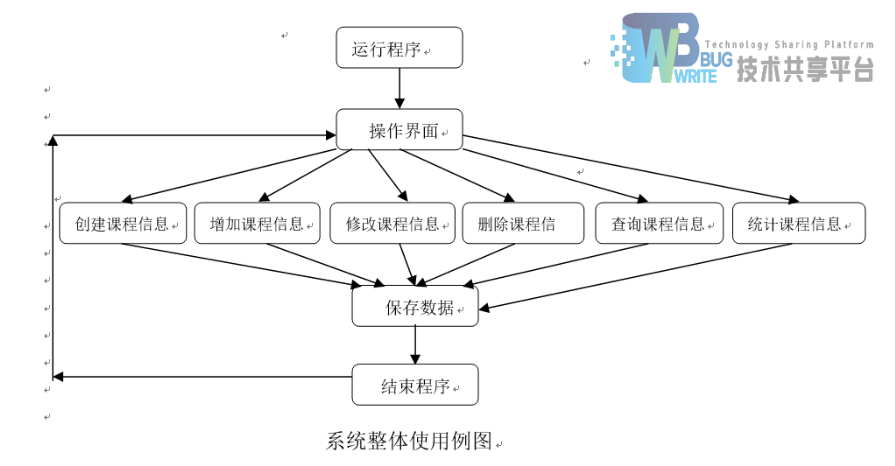点击此处下载文档和源码

展开全文• * 验证课程名称是否唯一 * true --- 不唯一 * @param name * @return */ public boolean name(String name) { boolean flag = false ; String sql = "select name from course where name = '...
• jsp+Servlet+Oracle 学生课程信息管理，内含数据库，源代码。 毕业设计，课程设计都可用！ 直接将 .dmp文件附加到oracle数据库即可发布使用！功能实用！欢迎下载！
• 系统基于vue+element-UI搭建，配合面试题库中后端服务开发，具有学生信息管理、课程信息管理、选课表管理、学生选课等多种功能。将功能区域划分为组件，数据处理迅速，面试题目中要求的所有功能皆已实现，并提供......